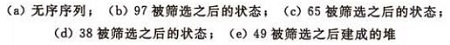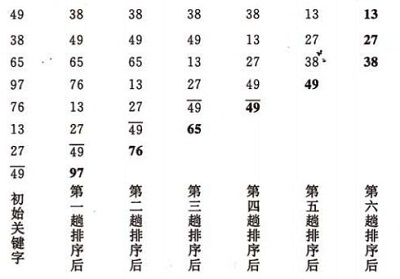# C/C++实现八大排序算法汇总

更新时间：2016年09月12日 09:50:39   作者：ywcpig1. 插入排序―直接插入排序(Straight Insertion Sort)```//插入排序
void Insert_Sort(int *list,int count)
{
int temp;/*此处充当哨兵，不在list数组里面单独占一个单位*/
int i,j;
for(i=1;i<count;i++)
{
if(list[i]<list[i-1])
{
temp = list[i];
for(j=i-1;list[j]>temp&&j>=0;j--)
{
list[j+1] = list[j];
}
list[j+1] = temp;
}
}
}

```

2. 插入排序―希尔排序（Shell`s Sort）```//shell排序
void Shell_Sort(int *list,int count)
{
int i,j;
int temp;
int increment = count;
do
{
increment = increment/2;
for(i = increment;i<count;i++)
{
if(list[i]<list[i-increment])
{
temp = list[i];
for(j=i-increment;j>=0&&list[j]>temp;j-=increment)
{
list[j+increment] = list[j];
}
list[j+increment] = temp;
}

}

}while(increment>1);
}

```

3. 选择排序―简单选择排序（Simple Selection Sort）```//选择排序
void Select_Sort(int *list,int count)
{
int min,i,j;
for(i=0;i<count;i++)
{
min = i;
for(j=i+1;j<count;j++)
{
if(list[min]>list[j])
{
min = j;
}
}
if(min!=i)
{
swap(list[i],list[min]);
}
}
}

```

4. 选择排序―堆排序（Heap Sort）（a）大顶堆序列：（96，83，27，38，11，09)

(b)  小顶堆序列：（12，36，24，85，47，30，53，91）1. 如何将n 个待排序的数建成堆；
2. 输出堆顶元素后，怎样调整剩余n-1 个元素，使其成为一个新堆。

1）设有m 个元素的堆，输出堆顶元素后，剩下m-1 个元素。将堆底元素送入堆顶（（最后一个元素与堆顶进行交换），堆被破坏，其原因仅是根结点不满足堆的性质。

2）将根结点与左、右子树中较小元素的进行交换。

3）若与左子树交换：如果左子树堆被破坏，即左子树的根结点不满足堆的性质，则重复方法 （2）.

4）若与右子树交换，如果右子树堆被破坏，即右子树的根结点不满足堆的性质。则重复方法 （2）.

5）继续对不满足堆性质的子树进行上述交换操作，直到叶子结点，堆被建成。1）n 个结点的完全二叉树，则最后一个结点是第个结点的子树。

2）筛选从第个结点为根的子树开始，该子树成为堆。

3）之后向前依次对各结点为根的子树进行筛选，使之成为堆，直到根结点。算法的实现：

```//调整为一个堆
{
int temp = list[s];
for(int j=2*s+1;j<=m;j = 2*j+1)
{
if(list[j]<list[j+1]&&j<m)
{
j++;
}
if(temp>list[j])
break;
list[s] = list[j];
s = j;
}
list[s] = temp;
}

//堆排序
void Heap_Sort(int *list,int len)
{
//创建一个大顶堆
for(int s = len/2-1;s>=0;s--)
{
}

//排序
for(int i = len-1;i >= 1;i--)
{
swap(list,list[i]);
}
}
```5. 交换排序―冒泡排序（Bubble Sort）```//冒泡排序
void Bubble_Sort(int *list,int count)
{
int flag = true;
int i = 0,j = 0;
for(i=0;i<=count&&flag;i++)
{
flag = false;
for(j=count -1;j>=i;j--)
{
if(list[j]<list[j-1])
{
swap(list[j],list[j-1]);
flag = true;
}
}
}
}

```

1．设置一标志性变量pos,用于记录每趟排序中最后一次进行交换的位置。由于pos位置之后的记录均已交换到位,故在进行下一趟排序时只要扫描到pos位置即可。

2．传统冒泡排序中每一趟排序操作只能找到一个最大值或最小值,我们考虑利用在每趟排序中进行正向和反向两遍冒泡的方法一次可以得到两个最终值(最大者和最小者) , 从而使排序趟数几乎减少了一半。

6. 交换排序―快速排序（Quick Sort）

（a）一趟排序的过程：```//快速排序
int Partition(int *list,int low,int high)
{
int pivotKey;
pivotKey = list[low];
while(low<high)
{
while(low<high&&list[high]>=pivotKey)
{
high--;
}
swap(list[low],list[high]);
while(low<high&&list[low]<=pivotKey)
{
low++;
}
swap(list[low],list[high]);
}
return low;
}

void Qsort(int *list,int low,int high)
{
int pivot;
if(low<high)
{
pivot =Partition(list,low,high);
Qsort(list,low,pivot-1);
Qsort(list,pivot+1,high);
}
}

void Quick_Sort(int *list,int count)
{
Qsort(list,0,count-1);
}
```

7. 归并排序（Merge Sort）1.j=m+1；k=i；i=i; //置两个子表的起始下标及辅助数组的起始下标

2.若i>m 或j>n，转⑷ //其中一个子表已合并完，比较选取结束

3.//选取r[i]和r[j]较小的存入辅助数组rf

4.//将尚未处理完的子表中元素存入rf

5.合并结束。

```//归并排序
//将两个有序数组排序
void Merge(int *list,int start,int mid,int end)
{
const int len1 = mid -start +1;
const int len2 = end -mid;
const int len = end - start +1;
int i,j,k;

int * front = (int *)malloc(sizeof(int)*len1);
int * back = (int *)malloc(sizeof(int)*len2);

for(i=0;i<len1;i++)
front[i] = list[start+i];
for(j=0;j<len2;j++)
back[j] = list[mid+j+1];

for(i=0,j=0,k=start;i<len1&&j<len2&&k<end;k++)
{
if(front[i]<back[j])
{
list[k] = front[i];
i++;
}else
{
list[k] = back[j];
j++;
}
}
while(i<len1)
{
list[k++] = front[i++];
}
while(j<len2)
{
list[k++] = back[j++];
}
}

void MSort(int *list,int start,int end)
{
if(start<end)
{
int mid = (start+end)/2;
MSort(list,0,mid);
MSort(list,mid+1,end);
Merge(list,start,mid,end);
}

}

void Merge_Sort(int *list,int count)
{
MSort(list,0,count-1);
}
```

1）首先是空间复杂度比较高，需要的额外开销大。排序有两个数组的空间开销，一个存放待排序数组，一个就是所谓的桶，比如待排序值是从0到m-1，那就需要m个桶，这个桶数组就要至少m个空间。

2）其次待排序的元素都要在一定的范围内等等。1）先按k1 排序分组，将序列分成若干子序列，同一组序列的记录中，关键码k1 相等。

2）再对各组按k2 排序分成子组，之后，对后面的关键码继续这样的排序分组，直到按最次位关键码kd 对各子组排序后。

3）再将各组连接起来，便得到一个有序序列。扑克牌按花色、面值排序中介绍的方法一即是MSD 法。

1) 先从kd 开始排序，再对kd-1进行排序，依次重复，直到按k1排序分组分成最小的子序列后。

2) 最后将各个子序列连接起来，便可得到一个有序的序列, 扑克牌按花色、面值排序中介绍的方法二即是LSD 法。

“多关键字排序”的思想实现“单关键字排序”。对数字型或字符型的单关键字，可以看作由多个数位或多个字符构成的多关键字，此时可以采用“分配-收集”的方法进行排序，这一过程称作基数排序法，其中每个数字或字符可能的取值个数称为基数。比如，扑克牌的花色基数为4，面值基数为13。在整理扑克牌时，既可以先按花色整理，也可以先按面值整理。按花色整理时，先按红、黑、方、花的顺序分成4摞（分配），再按此顺序再叠放在一起（收集），然后按面值的顺序分成13摞（分配），再按此顺序叠放在一起（收集），如此进行二次分配和收集即可将扑克牌排列有序。

```#define MAX 20
#define BASE 10

void Radix_Sort(int *a, int n) {
int i, b[MAX], m = a, exp = 1;

for (i = 1; i < n; i++) {
if (a[i] > m) {
m = a[i];
}
}

while (m / exp > 0) {
int bucket[BASE] = { 0 };

for (i = 0; i < n; i++) {
bucket[(a[i] / exp) % BASE]++;
}

for (i = 1; i < BASE; i++) {
bucket[i] += bucket[i - 1];
}

for (i = n - 1; i >= 0; i--) {
b[--bucket[(a[i] / exp) % BASE]] = a[i];
}

for (i = 0; i < n; i++) {
a[i] = b[i];
}

exp *= BASE;
}
}

```(1)平方阶(O(n2))排序

(2)线性对数阶(O(nlog2n))排序

(3)O(n1+§))排序,§是介于0和1之间的常数。

(4)线性阶(O(n))排序

1．待排序的记录数目n的大小；

2．记录本身数据量的大小，也就是记录中除关键字外的其他信息量的大小；

3．关键字的结构及其分布情况；

4．对排序稳定性的要求。

1）当n较大，则应采用时间复杂度为O(nlog2n)的排序方法：快速排序、堆排序或归并排序序。

2）当n较大，内存空间允许，且要求稳定性 =》归并排序

3）当n较小，可采用直接插入或直接选择排序。

5）一般不使用或不直接使用传统的冒泡排序。

6）基数排序

1、关键字可分解。
2、记录的关键字位数较少，如果密集更好
3、如果是数字时，最好是无符号的，否则将增加相应的映射复杂度，可先将其正负分开排序。